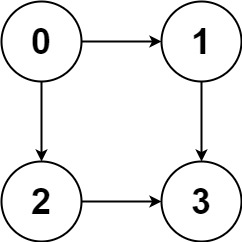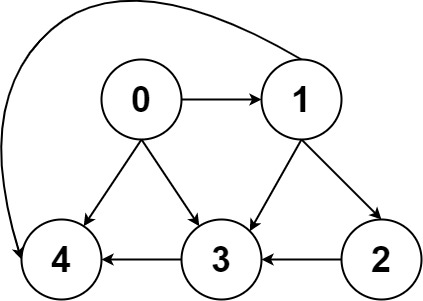| English | 简体中文 |

# 797. All Paths From Source to Target

## Description

Given a directed acyclic graph (DAG) of n nodes labeled from 0 to n - 1, find all possible paths from node 0 to node n - 1 and return them in any order.

The graph is given as follows: graph[i] is a list of all nodes you can visit from node i (i.e., there is a directed edge from node i to node graph[i][j]).

Example 1:Input: graph = [[1,2],,,[]]
Output: [[0,1,3],[0,2,3]]
Explanation: There are two paths: 0 -> 1 -> 3 and 0 -> 2 -> 3.


Example 2:Input: graph = [[4,3,1],[3,2,4],,,[]]
Output: [[0,4],[0,3,4],[0,1,3,4],[0,1,2,3,4],[0,1,4]]


Constraints:

• n == graph.length
• 2 <= n <= 15
• 0 <= graph[i][j] < n
• graph[i][j] != i (i.e., there will be no self-loops).
• All the elements of graph[i] are unique.
• The input graph is guaranteed to be a DAG.